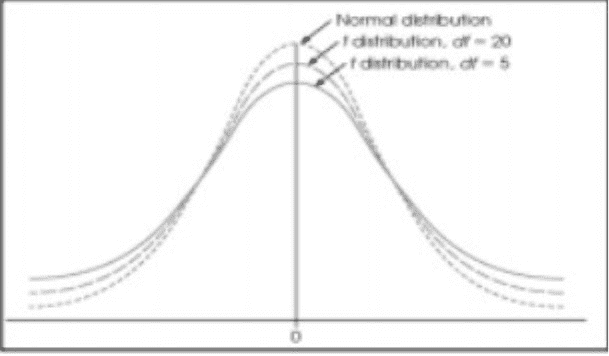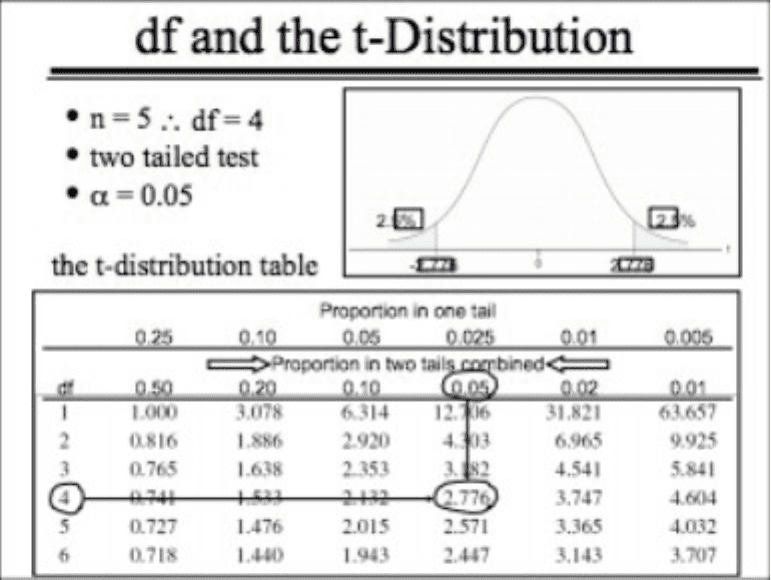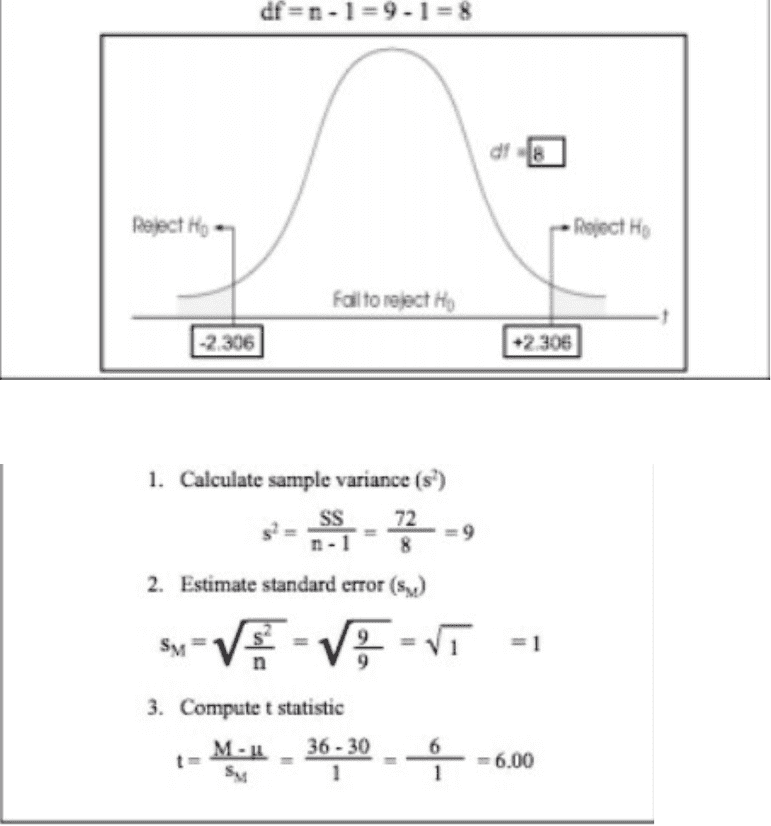Class Notes (1,000,000)
CA (610,000)
York (40,000)
PSYC (5,000)
Lecture 9

PSYC 2021 Lecture Notes - Lecture 9: Variance

Department
Psychology
Course Code
PSYC 2021
Professor
Alistair Mapp
Lecture
9

This preview shows pages 1-2. to view the full 8 pages of the document.November 19, 2015
Hypothesis Testing Review:
- M (sample mean) is an unbiased estimator of μ (population mean) //
this statement means that on average, the average sample mean will
be equal to the population mean
- σM speci%es the di&erence between M and μ due to chance
- z-score test quanti%es inferences about μ
z = M- μ / σM
= obtained di&erence between data H0 / expected di&erence due to
chance.
- for normally distributed z-scores, use the unit normal table to
determine critical z required to reject H0
z vs. t
- to use z, population variance (σ2) must be known to calculate
standard error (σM)
z = M- μ / σM
-more often than not, σ2 is unknown
-solution  use sample variance (s2) to estimate (σ2)
S2 = ss/n-1
-then use s2 to estimate standard error
SM = √s2/n
-To test hypotheses when σ2 is unknown use t-test
t = m – μ / SM
-Determine critical value of t using the t distribution table
Df and the t-distribution
- degrees of freedom (df)  the number of scores in a sample that are
free to vary
df= n-1
- unlike the z-distribution (always normally distributed), the shape of
the t-distribution varies as a function of df

Only pages 1-2 are available for preview. Some parts have been intentionally blurred.As sample size goes does, so does degrees of freedom and the curve
Use t because you don’t know the population variance
Using the t-distribution table: - df
- one tailed (predicting direction whether there is an increase or
decrease) or two tailed test
-α (will tell us the alpha level)
-
Hypothesis Testing with T
Example: Research Question – Does exposure to eye-spot patterns
a&ect the behaviour of moth-eating birds.
Procedure and results:
n= 9
time = 60 min
Dependent Variable = time in plain side
M = 36 min
SS = 72
Step 1: State the hypothesis about the unknown population
Null hypothesis  the treatment has no e&ect
H0 = μ plain side = 30 min
Alternative hypothesis  the treatment has an e&ect
H1: μ plain side ≠ 30 min

Unlock to view full version

Only pages 1-2 are available for preview. Some parts have been intentionally blurred.Step 2: Located the critical region based on a 2-tailed test, α = 0.05
Step 3: Calculate the test statistic (t)
Step 4: Make a decision